# uitree

Create tree or check box tree component

## Syntax

``t = uitree``
``t = uitree(style)``
``t = uitree(parent)``
``t = uitree(parent,style)``
``t = uitree(___,Name,Value)``

## Description

````t = uitree` creates a standard tree in a new figure window and returns the `Tree` object. MATLAB® calls the `uifigure` function to create the figure.```
````t = uitree(style)` creates a tree of the specified style. Specify `style` as `'checkbox'` to create a check box tree instead of a standard one.```

example

````t = uitree(parent)` creates a standard tree in the specified parent container. The parent can be a `Figure` created by using the `uifigure` function, or one of its child containers.```

example

````t = uitree(parent,style)` creates a tree of the specified style in the specified parent container.```

example

````t = uitree(___,Name,Value)` creates a tree with properties specified by one or more `Name,Value` arguments. Use this option with any of the input argument combinations in the previous syntaxes.```

## Examples

collapse all

Create a tree that contains a parent node called ```Sample Data``` with a child node called `Sample 1`. Expand the tree to see both nodes.

```fig = uifigure; t = uitree(fig); parent = uitreenode(t,'Text','Sample Data'); child = uitreenode(parent,'Text','Sample 1'); expand(t)```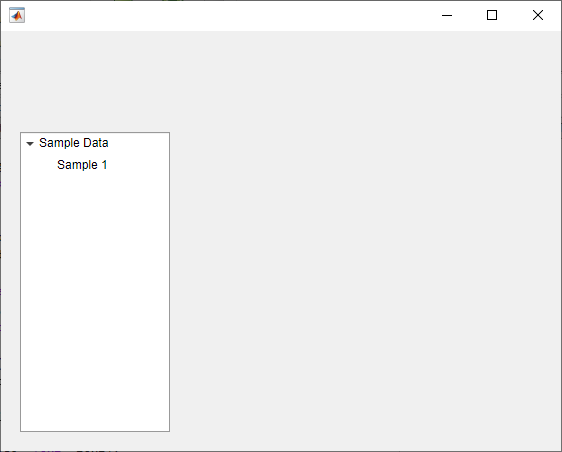Create a check box tree that contains a parent node called `Sample Data` with a child node called `Sample 1`. Expand the tree to see both nodes.

```fig = uifigure; t = uitree(fig,'checkbox'); parent = uitreenode(t,'Text','Sample Data'); child = uitreenode(parent,'Text','Sample 1'); expand(t)```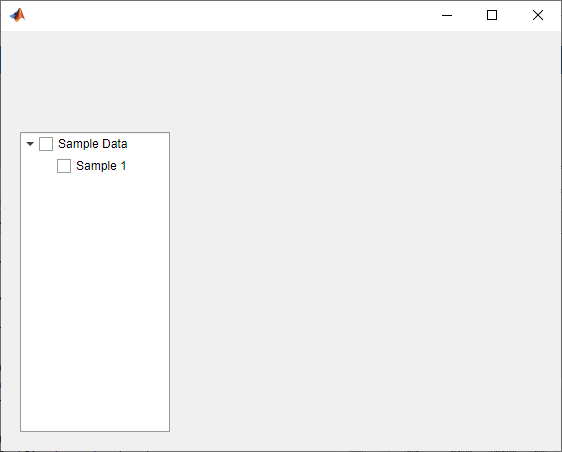Style nodes in a tree that showcases a file structure to visually distinguish different file types.

Create a tree UI component. Each top-level node represents a folder. Each child node represents a file in that folder. Expand the tree to see all the nodes.

```fig = uifigure("Position",[300 300 350 400]); t = uitree(fig); % Parent nodes n1 = uitreenode(t,"Text","App 1"); n2 = uitreenode(t,"Text","App 2"); n3 = uitreenode(t,"Text","Images"); % Child nodes n11 = uitreenode(n1,"Text","myapp1.m"); n21 = uitreenode(n2,"Text","myapp2.m"); n22 = uitreenode(n2,"Text","app2callback.m"); n31 = uitreenode(n3,"Text","peppers.png"); expand(t)```Create three styles: one with a bold font weight, one with an italic font angle, and one with an icon.

```dirStyle = uistyle("FontWeight","bold"); mStyle = uistyle("FontAngle","italic"); imgStyle = uistyle("Icon","peppers.png");```

Apply the bold style to the top-level nodes to distinguish the nodes that represent folders. Apply the italic style to the children of the ```App 1``` and `App 2` nodes to distinguish the nodes that represent MATLAB program files. Finally, apply the icon style to the node that represents an image file to show a preview of the image.

```addStyle(t,dirStyle,"level",1) addStyle(t,mStyle,"node",[n1.Children;n2.Children]) addStyle(t,imgStyle,"node",n31)```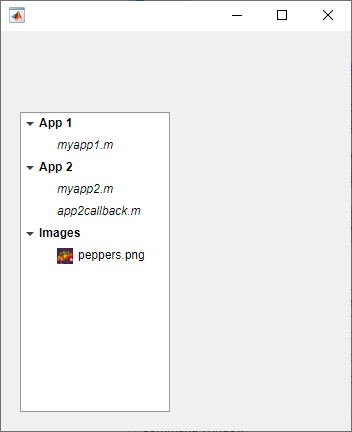Create an app that displays athlete names grouped by sport. When the app user clicks on a name, MATLAB displays data about the athlete.

Create a program file called `mytreeapp.m` that contains the following commands to create a tree, a set of nested tree nodes, and a callback function for the tree. The `SelectionChangedFcn` property specifies the function to execute when the user clicks a node in the tree.

```function mytreeapp fig = uifigure; t = uitree(fig,"Position",[20 20 150 150]); % Assign callback in response to node selection t.SelectionChangedFcn = @nodechange; % First level nodes category1 = uitreenode(t,"Text","Runners","NodeData",[]); category2 = uitreenode(t,"Text","Cyclists","NodeData",[]); % Second level nodes. % Node data is age (y), height (m), weight (kg) p1 = uitreenode(category1,"Text","Joe","NodeData",[40 1.67 58] ); p2 = uitreenode(category1,"Text","Linda","NodeData",[49 1.83 90]); p3 = uitreenode(category2,"Text","Rajeev","NodeData",[25 1.47 53]); p4 = uitreenode(category2,"Text","Anne","NodeData",[88 1.92 100]); % Expand the tree expand(t); % Create the function for the SelectionChangedFcn callback % When the function is executed, it displays the data of the selected item function nodechange(src,event) node = event.SelectedNodes; display(node.NodeData); end end```

When the user runs `mytreeapp` and clicks a node in the tree, MATLAB displays the `NodeData` for that node.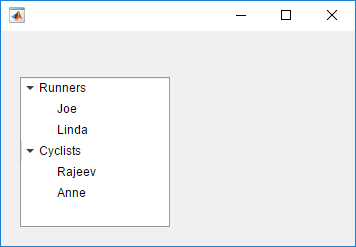Create an app that displays a grocery list grouped by food category. The app user can check individual items or entire food categories, and MATLAB displays the total weight of the checked items.

Create a program file called `mycheckboxtreeapp.m` that contains the following commands to create a check box tree, a set of nested tree nodes, and two callback functions for the check box tree. The `CheckedNodesChangedFcn` property specifies the function to execute when the user checks or unchecks a node in the tree. The `SelectedNodesChangedFcn` property specifies the function to execute when the user selects a node in the tree.

```function mycheckboxtreeapp fig = uifigure; cbt = uitree(fig,'checkbox','Position',[20 20 150 150]); % Assign callbacks in response to node check and selection cbt.CheckedNodesChangedFcn = @checkchange; cbt.SelectionChangedFcn = @selectchange; % First level nodes category1 = uitreenode(cbt,'Text','Vegetables','NodeData',[]); category2 = uitreenode(cbt,'Text','Fruits','NodeData',[]); % Second level nodes. % Node data is the weight of the food item (in grams) p1 = uitreenode(category1,'Text','Cucumber','NodeData',400); p2 = uitreenode(category1,'Text','Carrot','NodeData',65); p3 = uitreenode(category2,'Text','Apple','NodeData',183); p4 = uitreenode(category2,'Text','Banana','NodeData',120); % Expand the tree expand(cbt); % Create the function for the CheckedNodesChangedFcn callback % When this function is executed, it displays the total weight % of all checked items function checkchange(src,event) nodes = event.LeafCheckedNodes; if ~isempty(nodes) data = [nodes.NodeData]; display(sum(data)); end end % Create the function for the SelectedNodesChangedFcn callback % When this function is executed, it displays the name % of the selected item function selectchange(src,event) node = event.SelectedNodes; display(node.Text); end end```

When the user runs `mycheckboxtreeapp` and checks or unchecks a node in the tree, MATLAB displays the sum of the weights (stored in `NodeData`) for all the second-level checked nodes. When the user selects a node in the tree, MATLAB displays the text of that node.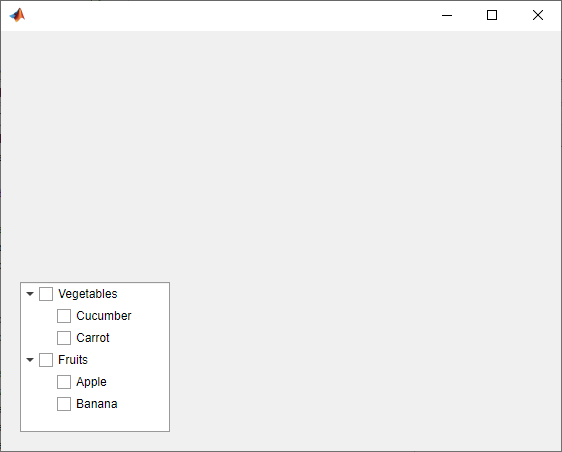Create a tree that populates nodes based on the data in a table.

Create a figure with a grid layout manager to hold the UI components. Load sample data on electric utility outages and create a table UI component to display the data. Then, create a tree to hold nodes listing the regions and causes of the outages.

```fig = uifigure; gl = uigridlayout(fig,[1 2]); gl.ColumnWidth = {'2x','1x'}; T = readtable("outages.csv"); T = T(1:20,["Region","OutageTime","Loss","Cause"]); tbl = uitable(gl,"Data",T); tr = uitree(gl);```

Specify the table variables to display in the tree. For each of those variables, create a top-level node whose text is the variable name. Extract the relevant data by converting the table entries for the variable to a categorical array and returning the list of categories as `names`. Then, loop through the categories. For each element, add a node to the tree under the appropriate parent node.

```vars = ["Region","Cause"]; for k1 = 1:length(vars) var = vars{k1}; varnode = uitreenode(tr,"Text",var); rows = T{:,var}; names = categories(categorical(rows)); for k2 = 1:length(names) text = names{k2}; uitreenode(varnode,"Text",text); end end```

Expand the tree to see all the nodes.

`expand(tr)`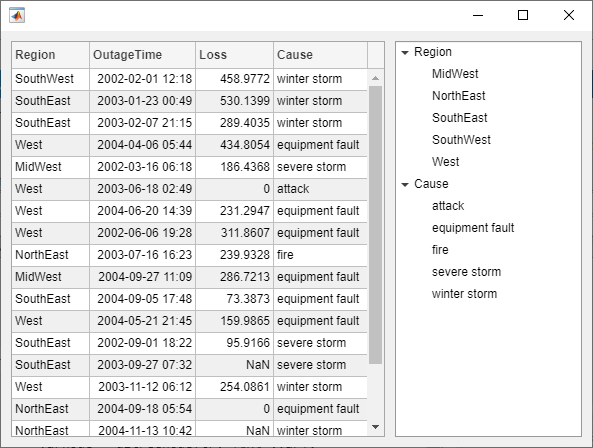## Input Arguments

collapse all

Style of tree, specified as one of the following:

• `'tree'` — Hierarchical list of items

• `'checkbox'` — Hierarchical list of items that can be checked, presented with a check box to the left of each item

Parent container, specified as a `Figure` object created using the `uifigure` function, or one of its child containers: `Tab`, `Panel`, `ButtonGroup`, or `GridLayout`. If you do not specify a parent container, MATLAB calls the `uifigure` function to create a new `Figure` object that serves as the parent container.

### Name-Value Arguments

Specify optional comma-separated pairs of `Name,Value` arguments. `Name` is the argument name and `Value` is the corresponding value. `Name` must appear inside single quotes (`' '`). You can specify several name and value pair arguments as `Name1,Value1,...,NameN,ValueN`.

Each type of `Tree` object supports a different set of properties. For a full list of properties and descriptions for each type, see the associated property page.

collapse all

### Selected Nodes

In a standard tree or a check box tree, a selected node is indicated by a blue highlight around the node text. The app user can select a node by clicking on the node text.

In a standard tree with the `Multiselect` property set to `'off'` and in every check box tree, at most one node can be selected at any time. In a standard tree, you can set the `Multiselect` property to `'on'` to allow for multiple nodes to be selected.

In this image, the `Carrot` node is selected.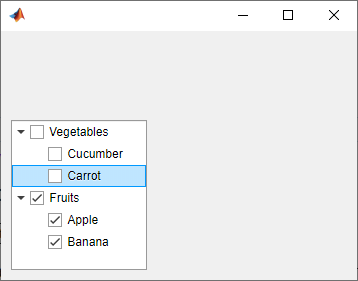### Checked Nodes

In a check box tree, a checked node is indicated by a checked check box to the left of the node text. Any number of nodes can be checked. The app user can check or uncheck a node by clicking on the check box. In a standard tree, you cannot check nodes.

In this image, the `Fruits`, `Apple`, and `Banana` nodes are checked.## Version History

Introduced in R2017b

expand all